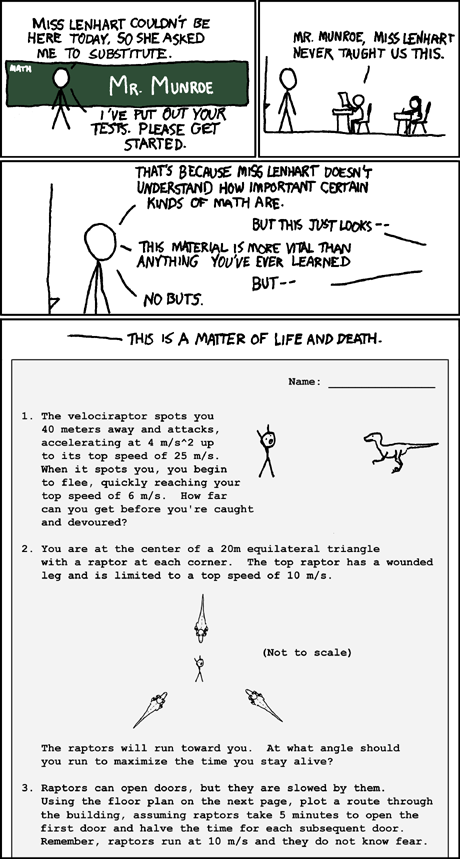# Clone of FOUNDATION MATHEMATICS

## Course Overview

Code:
LA Code:
Levels:
4
5
Learning Area:

LAs KayH, MatP, DunW, AliS, MatW,

In maths students will explore relationships in quantities, space and data and learn to express these relationships in ways that help them to make sense of the world around them.
Learning maths should help students to become knowledgeable citizens in an information and data driven time.
The fundamental learning outcomes in maths are to develop -
The ability to think creatively, critically and logically
The ability to structure and organize
The ability to process information
The ability to interpret and critically evaluate statistical information in a variety of contexts
The ability to solve problems that help them to investigate and understand the worldThere will be 5 classes in 2018 covering the curriculum at level 4 and 5. Across the year the following will be covered -

NUMBER

Use prime numbers, common factors, multiples powers and square roots.
Understand operations on fractions, decimals, percentages and integers
Use rates and ratios
Know commonly used fraction, decimal and percentage conversions
Know and apply standard form, significant figures, rounding and decimal place value

MEASUREMENT

Select and use appropriate metric units for length, area, volume and capacity, weight, temperature, angle and time
Convert between metric units using decimals
Deduce and use formulae to find the perimeters and areas of polygons and the volumes of prisms

Find the perimeters and areas of circles and composite shapes and the volumes of prisms including cylinders

ALGEBRA / GRAPHS

Form and solve linear and simple quadratic graphs
Generalise the properties of operations with fractional numbers and integers
Relate tables, graphs and equations to linear and simple quadratic relationships found in number and spatial patterns

STATISTICS

Plan and conduct surveys and experiments using the statistical enquiry cycle -
Determining appropriate variables and measures
Considering sources of variation
Gathering and cleaning data
Using multiple displays and re-categorising data to find patterns, variations, relationships and trends in multivariate data sets
Comparing sample distributions visually, using measures of centre, spread and proportion
Presenting a report of findings
Evaluate statistical investigations or probability activities undertaken by others, including data collection methods, choice of measures and validity of findings.

GEOMETRY

Deduce the angle properties of intersecting and parallel lines and the angle properties of of polygons and apply these properties
Create accurate nets for simple polyhedral and connect 3-D solids with different 2-D representations
Construct and describe simple loci
Interpret points and lines on co-ordinate planes, including scales and bearings on maps
Apply trigonometric ratios and Pythagoras’ theorem in two dimensions

PROBABILITY

Compare and describe the variation between theoretical and experimental distributions in situations that involve elements of chance
Calculate probabilities, using fractions, percentages and ratio

## Courses offered per block.

TitleLA CodeBlockYear